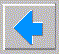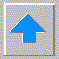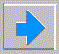# Lecture 7 - Predicate Logic and PROLOG

• proplog is wasteful since doesn’t have predicates or quantification
• there is another mathematically developed theory of logic - predicate logic
• Predicate Logic
• all of proplog and predicates, equality, quantifiers
• predicates (descriptions)
• round(ball)
• relations
• brother(isaac,ishmael)
• functions
• equality is now more useful
• abraham = father(isaac)
• quantification
• universal (ALL)
• existential (EXISTS)
• don’t need both due to DeMorgan relations
• all variables must be introduced with quantifier for wff
• PROLOG always assumes ALL
• the PROLOG programming language (CM)
• logic language - not procedural language
• no control structures - you never tell the computer what to do !
• no computational direction except
• depth first search
• backtracking when fail
• clause is parsed left to right
• clauses in knowledge base (KB) are considered in order of appearance
• standard CM Prolog gives a single answer, and waits - type ";" for the next answer
• elements of PROLOG
• constants (small letter), variables (capitals), structures (functor+components)
• facts: valuable(gold). female(mary). likes(john,mary). parent(abraham,isaac). gives(john,book,mary).
• variables: likes(john,X). means implicitly john likes all X
• anonymous variable _ .
• instantiation of variable (temporarily has value). var(X).
• questions
• ?- likes(john,mary). yes
• ?- likes(john,joan). no (means that can’t be proven from KB)
• ?- likes(john,X). X=mary
• implicit and
• ?- likes(john,mary), likes(john,joan).
• read "," as "and"
• X,Y succeeds iff X and Y do
• valuable(silver), valuable(gold).
• implicit or
• ?- likes(john,mary); likes(john,joan). yes
• read ";" as "or"
• ?- likes(john,X); likes(mary,X). X=mary
• rules
• mortal(X) :- human(X).
• read ":-" as "if"
• note implicit all
• FORALL X : a(X) ← b(X) is written in PROLOG a(X) :- b(X)
• mother(X,Y) :- female(X), parent(X,Y).
• parent(X,Y) :- father(X,Y); mother(X,Y).
• sister(X,Y) :- female(X), mother(M,X), mother(M,Y), father(F,X), father(F,Y), X\=Y.
• likes(john,X) :- female(X), likes(X,john).
• program examples - genesis family, Hanoi rings
• backtracking
• Prolog uses depth first search.
• Example: ?- grandfather(isaac, X).
• grandfather(X,Y) :- father(X,Z), parent(Z,Y). so instantiate X to isaac
• father(isaac,esau). so try Z = esau
• parent(esau,Y). means either father(esau,Y) or mother(esau,Y) - neither appears
• backtrack father(isaac,jacob). so try Z = jacob
• parent(jacob,Y). means either father(jacob,Y) or mother(jacob,Y)
• in order to appearance we find
• father(jacob,reuben).
• father(jacob,levi).
• father(jacob,judah).
• father(jacob,joseph).
• father(jacob,benjamin).
• so answers are reuben ; levi ; judah ; joseph; benjamin
• equality
• ?- X=Y. attempts to match X with Y.
• = is infix predicate that is predefined as X=X
• = will fail if sides are instantiated to different values
• = will succeed and instantiate if one side is a variable
• ?- john=john. yes
• ?- john=mary. no
• ?- X=john. X=john
• arithmetic
• most implementations are integer only
• is causes evaluation
• operators: > < >= =< \= + - * /
• program example - making change
• some built-in predicates
• true
• fail
• not(Clause)
• ! (cut)
• asserta(clause)
• assertz(clause)
• retract(clause)
• var(X)
• integer(X)
• atom(X)
• consult(file)
• listing(atom)
• get(Char)
• get0(Char)
• put(char)
• nl
• print(X)
• see(filename)
• seen
• tell(filename)
• told
• Example: Romanian cities